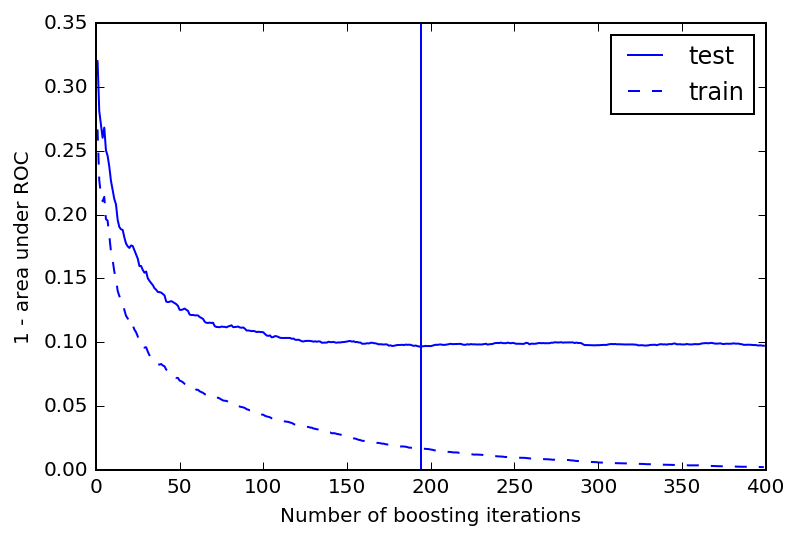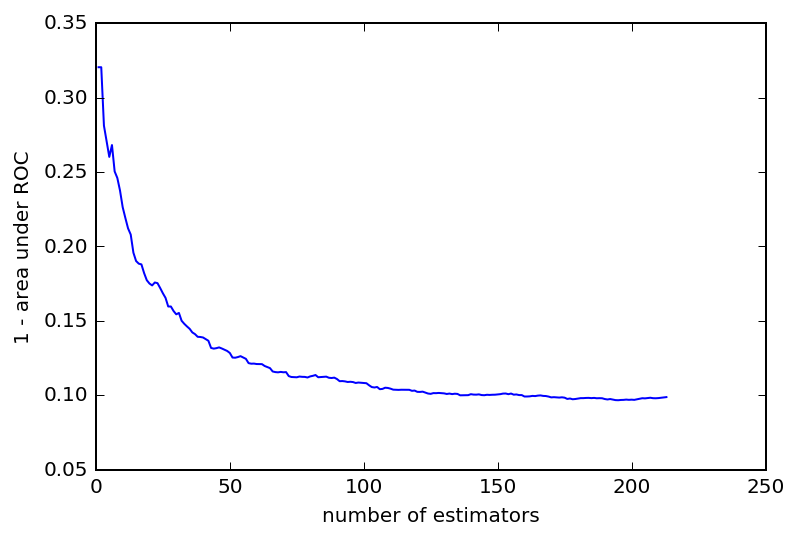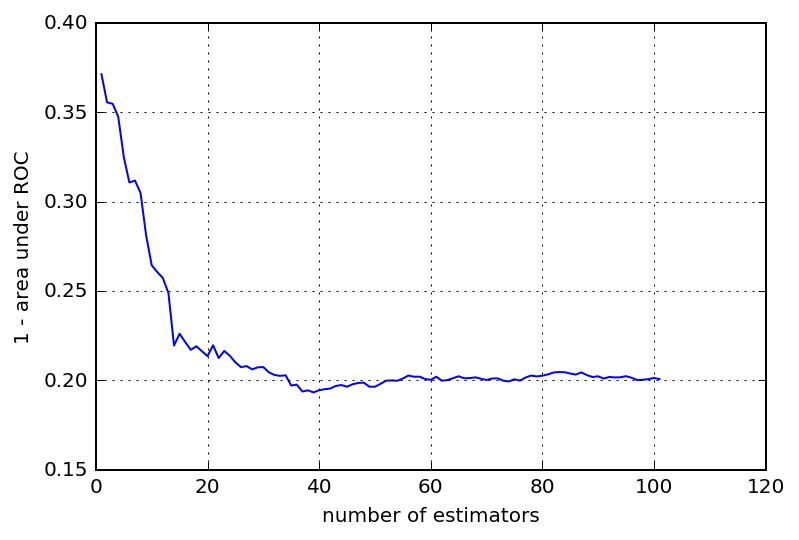# Stop growing your ensembles early

This is an interactive blog post, you can modify and run the code directly from your browser. To see any of the output you have to run each of the cells.

When building an ensemble of trees (a Random Forest or via gradient boosting) one question keeps coming up: how many weak learners should I add to my ensemble?

This post shows you how to keep growing your ensemble until the test error reaches a minimum. This means you do not end up wasting time waiting for your ensemble to build 1000 trees if you only need 200.

First a few imports and generating a toy dataset:

In :
%config InlineBackend.figure_format='retina'
%matplotlib inline

In :
import numpy as np

import matplotlib.pyplot as plt

from sklearn.cross_validation import train_test_split
from sklearn.datasets import make_classification
from sklearn.metrics import roc_auc_score

In :
X, y = make_classification(n_samples=4000,
n_features=40,
n_informative=10,
n_clusters_per_class=3,
random_state=2)
# Split data into a development and evaluation set
X_dev,X_eval, y_dev,y_eval = train_test_split(X, y,
train_size=0.5,
random_state=3)
# Split development set into a train and test set
X_train,X_test, y_train,y_test = train_test_split(X_dev, y_dev,
test_size=0.33, random_state=4)


## How many trees?¶

To get started we build an ensemble of 400 trees using gradient boosting. Without knowing how many trees are needed to reach the minimum test error we can just hope that 400 is enough. One downside is that we might be waiting a long time to fit a model with this many trees and then learn that we only needed a small fraction of them (say 200).

In :
opts = dict(max_depth=2, learning_rate=0.1, n_estimators=400)
_ = clf.fit(X_train, y_train)


Next we plot the validation curve for our fitted classifier and check with the test set at which number of n_estimators we reach the minimum test error.

In :
def validation_curve(clf):
test_score = np.empty(len(clf.estimators_))
train_score = np.empty(len(clf.estimators_))

for i, pred in enumerate(clf.staged_predict_proba(X_test)):
test_score[i] = 1-roc_auc_score(y_test, pred[:,1])

for i, pred in enumerate(clf.staged_predict_proba(X_train)):
train_score[i] = 1-roc_auc_score(y_train, pred[:,1])

best_iter = np.argmin(test_score)
test_line = plt.plot(test_score, label='test')

colour = test_line[-1].get_color()
plt.plot(train_score, '--', color=colour, label='train')

plt.xlabel("Number of boosting iterations")
plt.ylabel("1 - area under ROC")
plt.legend(loc='best')
plt.axvline(x=best_iter, color=colour)

validation_curve(clf)As suspected we reach the minimum after approximately 200 iterations. After this the score $1-\textrm{AUC}(\textrm{ROC})$ does not improve any further. In this case the performance does not degrade noticably with more iterations but it does take more time to build a model with 400 trees instead of 200.

Now that we know the answer let's see if we can construct a meta estimator that would have stopped at roughly 200 trees instead of fitting all 400.

## Early stopping¶

The obvious solution is to measure the performance of our ensemble as we go along and stop adding trees once we think we have reached the minimum.

The EarlyStopping meta estimator takes an unfitted estimator, the maximum number of iterations and a function to calculate the score as arguments.

It will repeatedly add one more base estimator to the ensemble, measure the performance, and check if we reached minimum. If we reached the minimum it stops, otherwise it keeps adding base estimators until it reaches the maximum number of iterations.

There are a few more details worth pointing out:

• there is a minimum number of trees required to skip over the noisier part of the score function; and

• EarlyStopping does not actually stop at the minimum, instead it continues on until the score has increased by scale above the current minimum. This is a simple solution to the problem that we only know we reached the minimum by seeing the score increase again.

In :
from sklearn.base import ClassifierMixin, clone
from functools import partial

def one_minus_roc(X, y, est):
pred = est.predict_proba(X)[:, 1]
return 1-roc_auc_score(y, pred)

class EarlyStopping(ClassifierMixin):
def __init__(self, estimator, max_n_estimators, scorer,
n_min_iterations=50, scale=1.02):
self.estimator = estimator
self.max_n_estimators = max_n_estimators
self.scorer = scorer
self.scale = scale
self.n_min_iterations = n_min_iterations

def _make_estimator(self, append=True):
"""Make and configure a copy of the estimator attribute.

Any estimator that has a warm_start option will work.
"""
estimator = clone(self.estimator)
estimator.n_estimators = 1
estimator.warm_start = True
return estimator

def fit(self, X, y):
"""Fit estimator using X and y as training set.

Fits up to max_n_estimators iterations and measures the performance
on a separate dataset using scorer
"""
est = self._make_estimator()
self.scores_ = []

for n_est in range(1, self.max_n_estimators+1):
est.n_estimators = n_est
est.fit(X,y)

score = self.scorer(est)
self.estimator_ = est
self.scores_.append(score)

if (n_est > self.n_min_iterations and
score > self.scale*np.min(self.scores_)):
return self

return self


We have all the ingredients, so let's take them for a spin. If EarlyStopping works it should stop adding trees to the ensemble before we get to 200 trees.

In :
def stop_early(classifier, **kwargs):
n_iterations = classifier.n_estimators
early = EarlyStopping(classifier,
max_n_estimators=n_iterations,
# fix the dataset used for testing by currying
scorer=partial(one_minus_roc, X_test, y_test),
**kwargs)
early.fit(X_train, y_train)
plt.plot(np.arange(1, len(early.scores_)+1),
early.scores_)
plt.xlabel("number of estimators")
plt.ylabel("1 - area under ROC")## Success ?!¶

Looks like it works. The EarlyStopping meta estimator suggests that around 210 trees is what is needed. While this is not in perfect agreement with what we found before, it is pretty good given how simple a "minimisation" strategy we use.

## Homework¶

Remember we split the dataset into three parts? We reserved one part as evaluation set. What is it good for? Should you quote the result from the test set as your best estimate of the performance of your classifier? Try and answer that question using the evaluation data set. Read my post on unbiased performance estimates if you need some inspiration.

## Taking if further¶

There are several ways to build on what we have created so far. Three I can think of:

1. try it with other types of estimators
2. better methods for dealing with the noise inherent to the score function
3. deal with very flat score functions

One and two are connected. Using EarlyStopping with a RandomForestClassifier works, however the score as a function of the number of estimators is much more noisy. Right now this means quite a bit of hand tuning of the minimum number of iterations and required increase (scale) to declare that we found a minimum.

To get you started, here a little snippet of using EarlyStopping with a random forest.

In :
from sklearn.ensemble import RandomForestClassifier

stop_early(RandomForestClassifier(n_estimators=400, max_depth=2),
n_min_iterations=100)
plt.grid()If you find a mistake or want to tell me something else get in touch on twitter @betatim

This post started life as a jupyter notebook, download it or view it online.